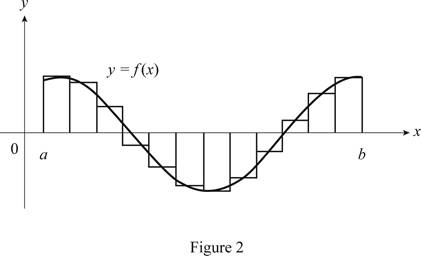# The expression for a Riemann sum of a function f .### Single Variable Calculus: Concepts...

4th Edition
James Stewart
Publisher: Cengage Learning
ISBN: 9781337687805### Single Variable Calculus: Concepts...

4th Edition
James Stewart
Publisher: Cengage Learning
ISBN: 9781337687805

#### Solutions

Chapter 5, Problem 1RCC

(a)

To determine

## To find: The expression for a Riemann sum of a function f.

Expert Solution

The expression for a Riemann sum of a function f is i=1nf(xi*)Δx.

### Explanation of Solution

The Riemann sum of a function f is the method to find the total area underneath a curve.

The area under the curve dividedas n number of approximating rectangles. Hence the Riemann sum of a function f is the sum of the area of the all individual rectangles.

R=i=1nf(xi*)Δx

Here, xi* is a point in the i subinterval [xi1,xi] and Δx is the length of the sub intervals.

Thus, the expression for a Riemann sum of a function f is i=1nf(xi*)Δx.

b)

To determine

Expert Solution

### Explanation of Solution

Given information:

Consider the condition for the function f(x)0

The function f(x)0 represents that the function is in the first quadrant of the graph.

Sketch the curve f(x) in the first quadrant and then separate the area under the curve with n number approximating rectangles.

Show the curve as in Figure 1.Refer to Figure 1

The function f(x) is positive. Hence the sum of areas of rectangles underneath the curve is the Riemann sum.

Thus, the geometric interpretation of a Riemann sum of f(x)0 is defined.

c)

To determine

Expert Solution

### Explanation of Solution

Given information:

The function f(x) takes on both positive and negative values.

The function f(x) takes on both positive and negative values represents that the function is in the first and fourth quadrant of the graph.

Sketch the curve f(x) in the first and third quadrant and then divide the area under the curve and above the curve with n number approximating rectangles.

Show the curve as in Figure 2.Refer figure 2,

The Riemann sum is the difference of areas of approximating rectangles above and below the x-axis

Therefore, the geometric interpretation of a Riemann sum is defined, if f(x) has both positive and negative values.

### Want to see more full solutions like this?

Subscribe now to access step-by-step solutions to millions of textbook problems written by subject matter experts!

### Have a homework question?

Subscribe to bartleby learn! Ask subject matter experts 30 homework questions each month. Plus, you’ll have access to millions of step-by-step textbook answers!